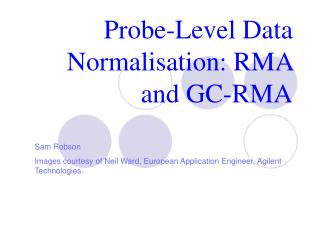DownloadDownload PresentationProbe-Level Data Normalisation: RMA and GC-RMA

# Probe-Level Data Normalisation: RMA and GC-RMA

Télécharger la présentation## Probe-Level Data Normalisation: RMA and GC-RMA

- - - - - - - - - - - - - - - - - - - - - - - - - - - E N D - - - - - - - - - - - - - - - - - - - - - - - - - - -
##### Presentation Transcript

1. Probe-Level Data Normalisation: RMA and GC-RMA Sam Robson Images courtesy of Neil Ward, European Application Engineer, Agilent Technologies.

2. References • “Summaries of Affymetrix Genechip Probe Level Data”, Irizarry et al., Nucleic Acids Research, 2003, Vol. 31, No. 4. • “Exploration, Normalization and Summaries of High Density Oligonucleotide Array Probe Level Data”, Irizarry et al., … • “A Model Based Background Adjustment for Oligonucleotide Expression Arrays”, Wu, Irizarry et al., Johns Hopkins University, Dept. of Biostatistics

3. Affymetrix Genechips • Each gene represented by 11-20 ‘probe pairs’. • Probe pairs are 3’ biased. • ‘Probe Pair’ consists of Perfect Match (PM) and MisMatch (MM) probes. • MM has altered middle (13th) base. Designed to measure non-specific binding (NSB).

4. Genechip Scanning • RNA sample prepared, labelled and hybridised to chip. • Chip fluorescently scanned. Gives a raw pixelated image - .DAT file. • Grid used to separate pixels related to individual probes. • Pixel intensities averaged to give single intensity for each probe - .CEL file. • Probe level intensities combined for each probe set to give single intensity value for each gene.

5. Affymetrix MicroArray Suite (MAS) v4.0 • Uses MM probes to correct for NSB. • MAS4.0 used simple Average Difference method: • A is the subset of probes where is within 3 SDs of the average of • Excludes outliers, but not a robust averaging method.

6. Affymetrix MicroArray Suite (MAS) v5.0 • Current method employed by Affymetrix. • Weighted mean using one-step Tukey Biweight Estimate: • CTj is a quantity derived from MMj never larger than PMj. • Weights each probe intensity based on it’s distance from the mean. • Robust average (insensitive to small changes from any assumptions made).

7. Tukey Biweight

8. Problems with Mis-Match data • MM intensity levels are greater than PM intensity levels in ~1/3 of all probes. • Suggests that MM probes measure actual signal, and not just NSB. • Removal of MM results in negative signal values. • Subtracting MM data will result in loss of interesting signal in many probes. Several methods have been proposed using only PM data.

9. Problems with Mis-Match data

10. Problems with MAS5.0 • Loss of probe-level information. • Background estimate may cause noise at low intensity levels due to subtraction of MM data.

11. Robust Multiarray Average (RMA) • Subtraction of MM data corrects for NSB, but introduces noise. • Want a method that gives positive intensity values. • Normalising at probe level avoids the loss of information.

12. Robust Multiarray Average (RMA) • Background correction. • Normalization (across arrays). • Probe level intensity calculation. • Probe set summarization.

13. Robust Multiarray Average (RMA) • PM data is combination of background and signal. • Assume strictly positive distribution for signal. Then background corrected signal is also positively distributed. • Background correction performed on each array seperately.

14. Robust Multiarray Average (RMA) • Background correction. • Normalization (across arrays). • Probe level intensity calculation. • Probe set summarization.

15. Robust Multiarray Average (RMA) • Normalises across all arrays to make all distributions the same. • ‘Quantile Normalization’ used to correct for array biases. • Compares expression levels between arrays for various quantiles. • Can view this on quantile-quantile plot. • Protects against outliers.

16. Robust Multiarray Average (RMA) • Background correction. • Normalization (across arrays). • Probe level intensity calculation. • Probe set summarization.

17. Robust Multiarray Average (RMA) • Linear model. • Uses background corrected, normalised, log transformed probe intensities (Yijn). μin=Log scale expression level (RMA measure). αjn = Probe affinity affect. εijn = Independent identically distributed error term (with mean 0).

18. Robust Multiarray Average (RMA) • Background correction. • Normalization (across arrays). • Probe level intensity calculation. • Probe set summarization.

19. Robust Multiarray Average (RMA) • Combine intensity values from the probes in the probe set to get a single intensity value for each gene. • Uses ‘Median Polishing’. • Each chip normalised to its median. • Each gene normalised to its median. • Repeated until medians converge. • Maximum of 5 iterations to prevent infinate loops.

20. Robust Multiarray Average (RMA) Pre-Normalisation

21. Robust Multiarray Average (RMA) Post-Normalisation

22. GC-RMA • Corrects for background noise as well as NSB. • Probe affinity calculated using position dependant base effects: • MM data adjusted based on probe affinity, then subtracted from PM. • Does not lose MM data.

23. Advantages of RMA/GC-RMA • Gives less false positives than MAS5.0. • See less variance at lower expression levels than MAS5.0. • Provides more consistent fold change estimates. • Exclusion of MM data in RMA reduces noise, but loses information. • Inclusion of adjusted MM data in GC-RMA reduces noise, and retains MM data.

24. Disadvantages of RMA/GC-RMA • May hide real changes, especially at low expression levels (false negatives). • Makes quality control after normalisation difficult. • Normalisation assumes equal distribution which may hide biological changes.

25. Conclusions • RMA is more precise than MAS5.0, but may result in false negatives at low expression levels. • Useful for fold change analysis, but not for studying statistical significance. Makes quality control difficult. • Ideal solution – Use standard MAS5.0 techniques for quality control. Then go back and perform probe level normalisation on quality controlled genes.